# How to convert Column Letter to Number in Excel

This post will guide you how to convert column letter to number in Excel. How do I convert letter to number with a formula in Excel. How to convert column letter to number with VBA macro in Excel. How to use a User Defined Function to convert letter to number in Excel.

## Convert Column Letter to Number with a Formula

If you want to convert a column letter to a regular number, and you can use a formula based on the COLUMN function and the INDIRECT function to achieve the result.

For example, you need to convert a column letter in Cell B1 to number

Just like this:

`=COLUMN(INDIRECT(B1&1))`

Type this formula into a blank cell such as: C1, and press Enter key, and then drag the AutoFill Handle over to other cells to apply this formula.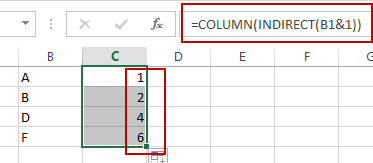The INDIRECT function will convert the text into a proper Excel reference and then pass the result to Column function to get the column number for the reference.

## Convert Column Letter to Number with VBA Macro

You can also use an Excel VBA Macro to achieve the result of converting column letter into its corresponding numeric value. Just do the following steps:

#1 open your excel workbook and then click on “Visual Basic” command under DEVELOPER Tab, or just press “ALT+F11” shortcut.#2 then the “Visual Basic Editor” window will appear.

#3 click “Insert” ->”Module” to create a new module.#4 paste the below VBA code into the code window. Then clicking “Save” button.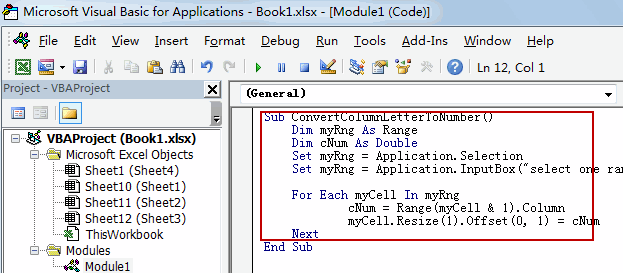```Sub ConvertColumnLetterToNumber()
Dim myRng As Range
Dim cNum As Double
Set myRng = Application.Selection
Set myRng = Application.InputBox("select one range that contain column letters", "ConvertColumnLetterToNumber", myRng.Address, Type:=8)

For Each myCell In myRng
cNum = Range(myCell & 1).Column
myCell.Resize(1).Offset(0, 1) = cNum
Next
End Sub```

#5 back to the current worksheet, then run the above excel macro. Click Run button.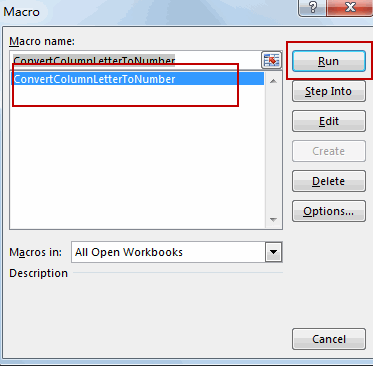#6 select one range that contain column letters, such as B1:B4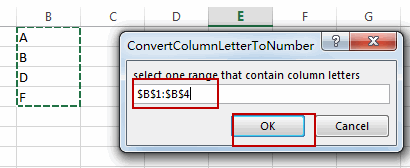#7 let’s see the result: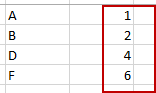## Convert Column Letter to Number with User Defined Function

Excel does not have a built in formula to convert column letter to a numeric number, but you can write down a User Defined Function to achieve the result. Just do the following steps:

#1 repeat the above step 1-3

#2 paste the below VBA code into the code window. Then clicking “Save” button.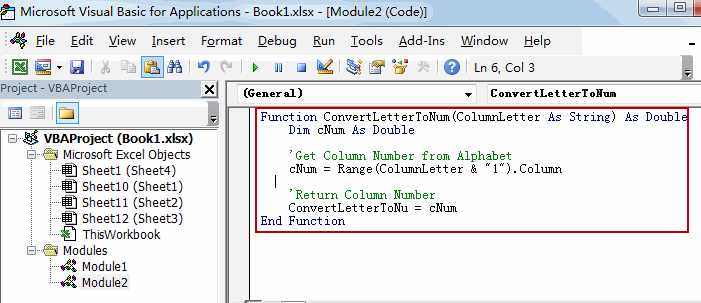```Function ConvertLetterToNum(ColumnLetter As String) As Double
Dim cNum As Double

'Get Column Number from Alphabet
cNum = Range(ColumnLetter & "1").Column

'Return Column Number
ConvertLetterToNum = cNum
End Function```

#3 back to the current worksheet, then type the following formula in a blank cell. press Enter key.

=ConvertLetterToNum(B1)

#4 select the cell C1, and  drag the AutoFill Handle over to other cells to apply this formula.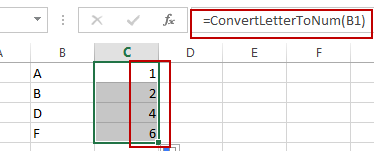## Convert Number to Column Letter

If you want to convert column number to an Excel Column letter, you can use another formula based on the SUBSTITUTE function and the ADDRESS function. Just like this:

`=SUBSTITUTE(ADDRESS(1,C1,4),"1","")`

Type this formula into Cell D1, and press Enter key. And then drag the AutoFill handle over to other cells to apply this formula.

Let’s see the last result: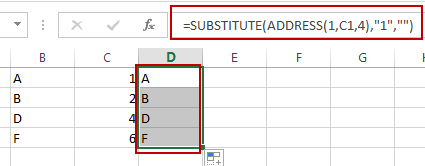Video: Convert Column Letter to Number

### Related Functions

• Excel Substitute function
The Excel SUBSTITUTE function replaces a new text string for an old text string in a text string.The syntax of the SUBSTITUTE function is as below:= SUBSTITUTE  (text, old_text, new_text,[instance_num])….
The Excel ADDRESS function returns a reference as a text string to a single cell.The syntax of the ADDRESS function is as below:=ADDRESS (row_num, column_num, [abs_num], [a1], [sheet_text])….
• Excel INDIRECT function
The Excel INDIRECT function returns the cell reference based on a text string, such as: type the text string “A2” in B1 cell, it just a text string, so you can use INDIRECT function to convert text string as cell reference….
• Excel COLUMN function
The Excel COLUMN function returns the first column number of the given cell reference.The syntax of the COLUMN function is as below:=COLUMN ([reference])….
Related Posts

Find and Replace Multiple Values

This post will guide you how to find and replace multiple values at once with VBA macro or using formula  in Excel. How do I make multiple find and replace in Excel. Suppose that you have a few cells containing ...

VBA Macro For VLOOKUP From Another Sheet

In the previous post, you should know that how to fix or remove the #N/A error when using VLOOKUP formula to lookup value from another sheet. And this post will show you how to use VBA code to vlookup data ...

How To Insert Comments in Protected Worksheet in Excel

This post will show you how to allow comments in a protected worksheet in Excel. You can easily to insert comments into cells in a normal worksheet in Excel, but if want to insert a comment in a worksheet that ...

How To Convert Text to Upper Cases(Using VBA) in Excel

This post will show you how to switch from lower case to upper case in Excel. and I am going to show you two different ways of converting text to upper cases using formula or VBA macro in Excel 2013,Excel ...

How To Hide Every Other Row in Excel (Using VBA)

This post will show you how to hide alternate rows or columns in Excel or how to hide every third, fourth, fifth row or column in Excel. If you want to hide every other row in your current worksheet, how ...

How to Disable the Save As Prompt in Excel

This post will show you how to use a VBA Macro to save an Excel file and overwrite any existing file without a prompt so that you are going to get the little window that says file already exists do ...

How to Count Cells that Contain even or odd numbers in Excel

This post will guide you how to count the number of cells that contain odd or even numbers within a range of cells using a formula in Excel 2013/2016.How do I count cells that contain odd numbers through the use ...

How to Count Cells that Contain negative Numbers in Excel

This post will guide you how to count the number of cells that contain negative numbers within a range of cells using a formula in Excel 2013/2016.You can count the number of negative numbers in your data using easy functions ...

How to Count Cells Are Not Blank or Empty in Excel

This post will guide you how to count cells that are not blank or empty in a given range cells using a formula in Excel 2013/2016.How do I count the number of cells that are not blank in a particular ...

How to Count Cells Less Than a Specific Value in Excel

This post will guide you how to count the number of cells less than a particular numeric value in a given range cells using a formula in Excel 2013/2016. How do I count cells that are less than a specific ...

Sidebar